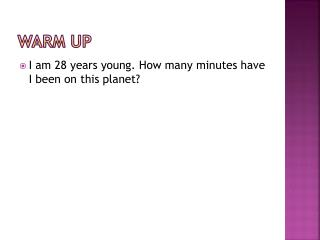Download PresentationWarm UP

# Warm UP - PowerPoint PPT PresentationDownload Presentation## Warm UP

- - - - - - - - - - - - - - - - - - - - - - - - - - - E N D - - - - - - - - - - - - - - - - - - - - - - - - - - -
##### Presentation Transcript

1. Warm UP • I am 28 years young. How many minutes have I been on this planet?

2. Dimensional analysis • A systematic method for solving problems in which units are carried through the entire process • Helps ensure that problems are solved correctly and have the proper units. • Uses conversion factors • a fraction whose numerator and denominator are equal quantities expressed in different units • used to change from one unit to another

3. Common Conversion Factors 1 inch = 2.54 cm 1 pound = 454 grams 1 quart = 0.946 liters 1 mile = 1.609 km 1 kilo = 1000 100 centi = 1 1000 milli = 1 1 cm3 = 1 mL

4. Examples • 25.0 hop = _________________hip • 8 hop = 3 hap • 1 hip = 6 hap • A lab bench is 175 inches long. What is its length in meters? • In France, Martha drove 195 km/hr. What was her speed in mi/hr?

5. Significant Figures in Calculations • Consider only measured numbers when determining the number of significant figures in an answer. • Ignore counted numbers • Ignore defined numbers

6. Examples • 25.0 hop = _________________hip • 8 hop = 3 hap • 1 hip = 6 hap • A lab bench is 175 inches long. What is its length in meters? • In France, Martha drove 195 km/hr. What was her speed in mi/hr?

7. Dimensional analysis stations Directions: Using the flashcards at each lab table, work the following conversion problems using the dimensional analysis method. Show all of your work and include the correct answer. Be sure to include units.  • Problem 1: Convert 7 orks to oops. • Problem 2: Convert 9.1 triangle’s to star’s. • Problem 3: Convert 12 A to F. • Problem 4: Convert 32 Do to La. • Problem 5: Convert 1.0 year to seconds. • Problem 6: Convert 60.25 mm/sec to km/hr.